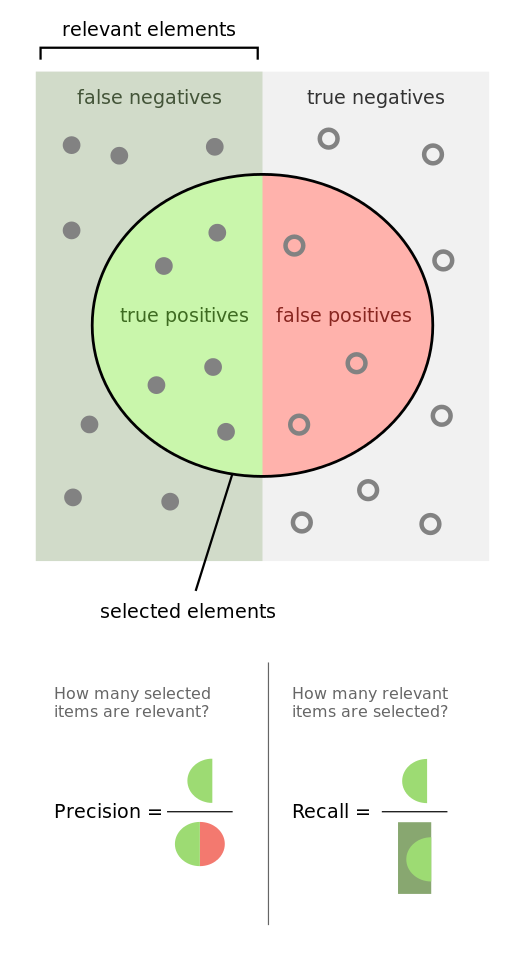# Logistic Regression

## Binary Classification

Reminder: Probabilities bounded between 0 and 1

Given a set of features, what is the probability that this data point belongs to $y = 1$ or $y = 0$?

If P[ y = 1 | x] > 0.5, then $\hat{y} = 1$, else $\hat{y} = 0$

### Probabilistic Interpretation

Linear regression returns any real number

What function can we apply to $z = w^Tx$ to bound it between 0 and 1?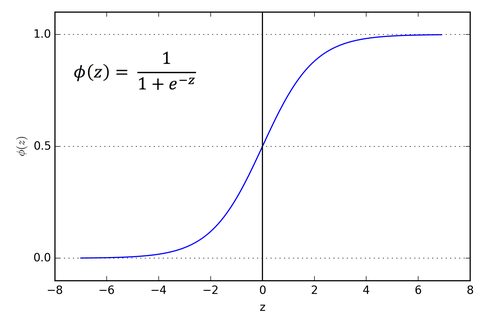## Logistic FunctionLarge positive inputs => 1

Large negative inputs => 0

## Make predictions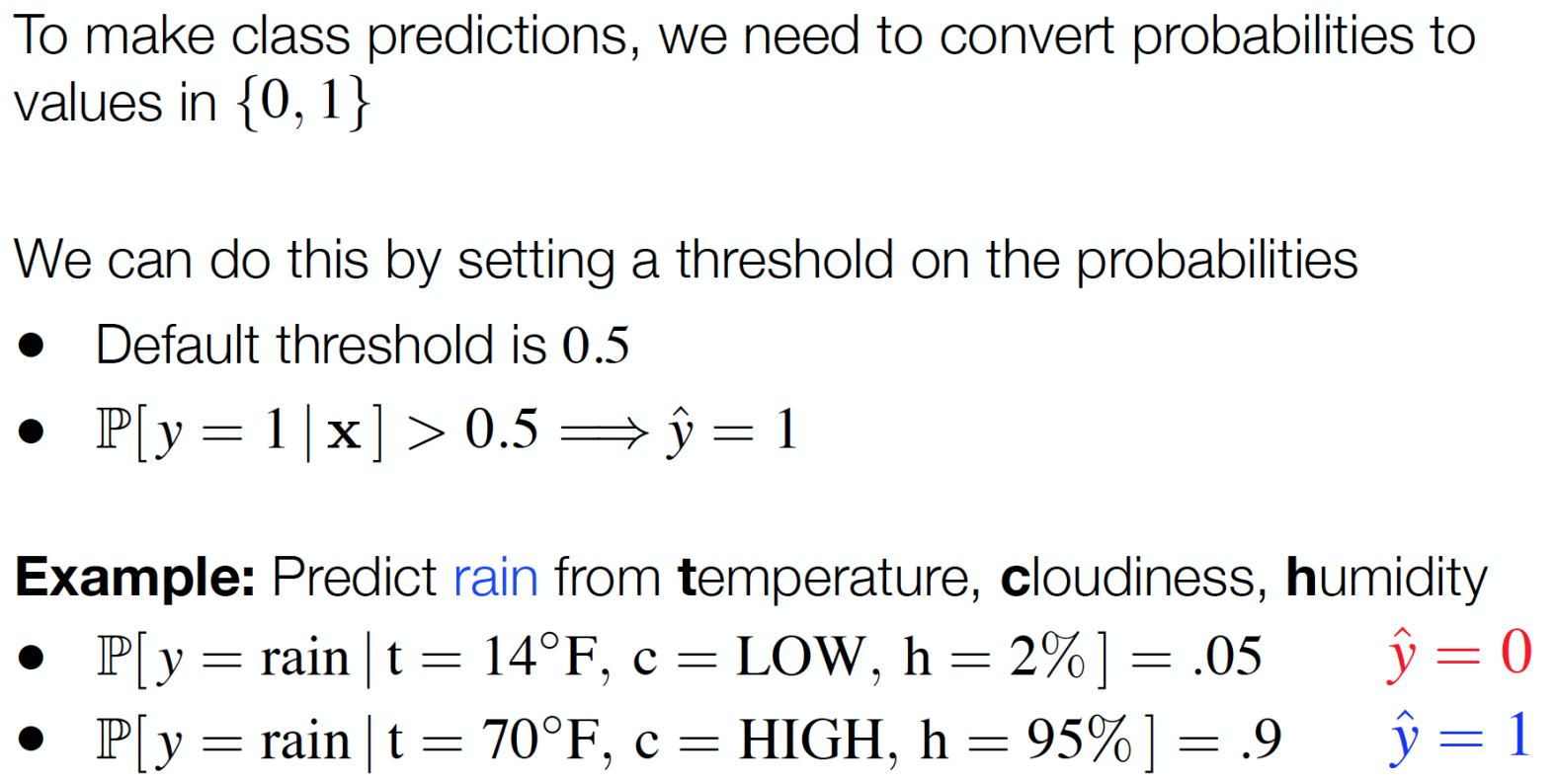## Confusion Matrix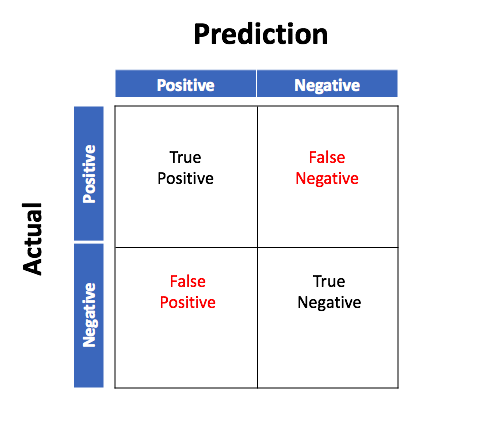## Types of Errors?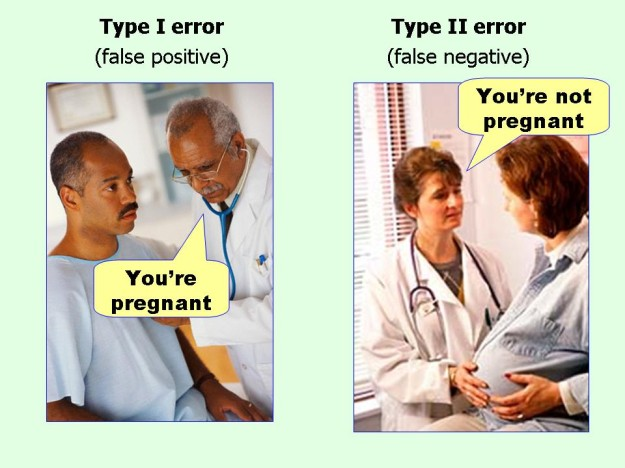## Alternative Metrics